# Excercise 6.4 Squares and Square Roots- NCERT Solutions Class 8

Go back to  'Squares and Square Roots'

## Chapter 6 Ex.6.4 Question 1

Find the square root of each of the following numbers by division method.

(i) $$2304$$

(ii) $$4489$$

(iii) $$3481$$

(iv) $$529$$

(v) $$3249$$

(vi) $$1369$$

(vii) $$5776$$

(viii) $$7921$$

(ix) $$576$$

(x) $$1024$$

(xi) $$900$$

(xii) $$900$$

### Solution

What is Known?

Perfect squares

What is unknown?

Square root by using division method.

Reasoning:

When a number is large, even the method of trading the square root by prime factorization becomes lengthy and difficult, so the division method is used.

Steps

(i)

The square root of $$2304$$ is calculated as follows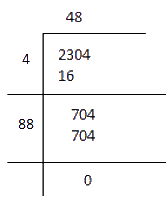Since remainder is zero and number of digits and left in the given number. Therefore,

$$\sqrt {2304}= 48$$

(ii)

The square root of $$4489$$ is calculated as follows.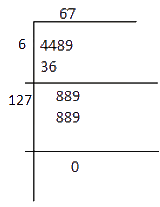\begin{align}\sqrt {4489} = 67\end{align}

(iii)

The square root of $$3481$$ is calculated as follows.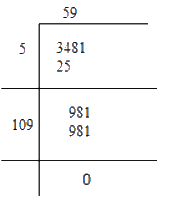$$\sqrt {3841}= 59$$

(iv)

The square root of $$529$$ is calculated as follows.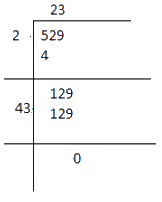$\sqrt {529}= 23$

(v)

The square root of $$3249$$ is calculated as follows.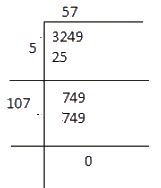$\sqrt {3249}= 57$

(vi)

The square root of $$1369$$ is calculated as follows.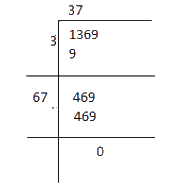$\sqrt {1369}= 37$

(vii)

The square root of $$5776$$ is calculated as follows.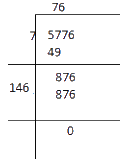$\sqrt {5776}= 146$

(viii)

The square root of $$7921$$ is calculated as follows.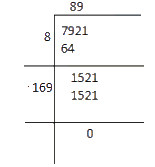$\sqrt {7921}= 89$

(ix)

The square root of $$576$$ is calculated as follows.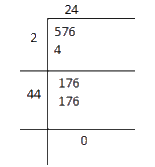$\sqrt {576}= 24$

(x)

The square root of $$1024$$ is calculated as follows.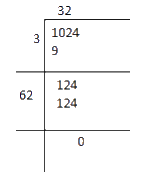$\sqrt {1024}= 32$

(xi)

The square root of $$3136$$ is calculated as follows.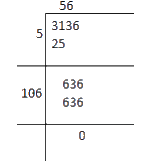$\sqrt {3136}= 56$

(xii)

The square root of $$900$$ is calculated as follows.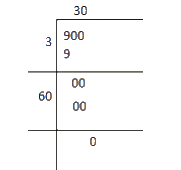$\sqrt {900}= 30$

## Chapter 6 Ex.6.4 Question 2

Find the number of digits in the square root of each of the following numbers (without any calculation).

(i) $$64$$

(ii) $$144$$

(iii) $$4489$$

(iv) $$27225$$

(v) $$390625$$

### Solution

What is known?

Squares

What is unknown?

Number of digits in square root

Reasoning:

If a perfect square is of n digits then it’s square root will have \begin{align}\frac{n}{2}\end{align} digits, if n is even and \begin{align}\frac{{\left( {n + 1} \right)}}{2}\end{align} if $$n$$ is odd

Steps

(i) $$64$$

$$n = 2 \rm(even)$$

\begin{align}{\rm{Number}}\;{\rm{of}}\;{\rm{digits}} = \frac{2}{2} = 1\end{align}

(ii) $$144$$

$$n = 3$$

\begin{align}{\rm{Number}}\;{\rm{of}}\;{\rm{digits}} &= \frac{{n + 1}}{2}\\ &= \frac{{3 + 1}}{2} \\&= \frac{4}{2} = 2\end{align}

(iii) $$4489$$

$$n = 4$$

\begin{align}{\rm{Number}}\;{\rm{of}}\;{\rm{digits}} = \frac{n}{2} = \frac{4}{2} = 2\end{align}

(iv) $$27225$$

$$n = 5$$

\begin{align}{\rm{Number}}\;{\rm{of}}\;{\rm{digits}} &= \frac{{n + 1}}{2}\\ &= \frac{{5 + 1}}{2} \\&= \frac{6}{2} = 3\end{align}

(v) $$390625$$

$$n = 6$$

\begin{align}{\rm{Number}}\;{\rm{of}}\;{\rm{digits}} = \frac{n}{2} = \frac{6}{2} = 3\end{align}

## Chapter 6 Ex.6.4 Question 3

Find the square root of the following decimal numbers.

(i) $$2.56$$

(ii) $$7.29$$

(iii) $$51.84$$

(iv) $$42.25$$

(v) $$31.36$$

### Solution

What is known?

Perfect squares in decimal form

What is unknown?

Square root by using division method

Reasoning:

To find the square root of decimal numbers put bars on the integral part of the number and place bars on the decimal part on every pair of digits starting with the first decimal place.

Steps:

(i)

Square root of $$2.56$$ is calculated as:-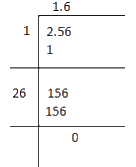$\sqrt {2.56}= 1.6$

(ii)

Square root of $$7.29$$ is calculated as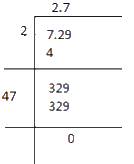$\sqrt {7.29}= 2.7$

(iii)

Square root of $$51.84$$ is calculated as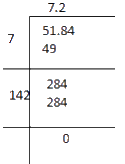$\sqrt {51.84}= 7.2$

(iv)

Square root of $$42.25$$ is calculated as follows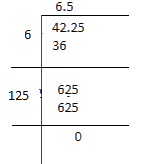$\sqrt {42.25}= 6.25$

(v)

Square root of $$31.36$$ is calculated as follows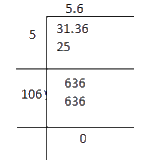$\sqrt {31.36}= 5.6$

## Chapter 6 Ex.6.4 Question 4

Find the least number which must be subtracted from each of the following numbers so as to get a perfect square. Also find the square root of the perfect square so obtained.

(i) $$402$$

(ii) $$1989$$

(iii) $$3250$$

(iv) $$825$$

(v) $$4000$$

### Solution

What is known?

Numbers that are not perfect square

What is unknown?

What must be subtracted from the numbers so as to get perfect square

Steps

(i)

Square root of $$402$$ is calculated as: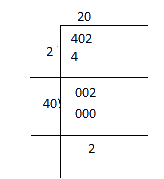It is evident that square of $$20$$ is less than $$402$$ by $$2$$. If we subtract the remainder from the number, we get a perfect square. Therefore, the required perfect square is

Therefore, required perfect square $$= 402 − 2 = 400$$

$$402 - 2 = 400\;{\rm{and}}\;\sqrt {400}= 20$$

(ii)

Square root of $$1989$$ by long division method.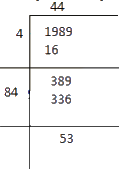The remainder obtained is $$53$$. The square of $$44$$ is less than the given number $$1989$$ by $$53$$. Therefore, perfect square can be obtained by subtracting $$53$$ from the given number $$1989$$.

Therefore, required perfect square $$= 1989 − 53 = 1936$$

$${\rm{And}}\;\sqrt {1936}= 44$$

(iii)

Square root of $$3250$$ can be calculated by long division method as follows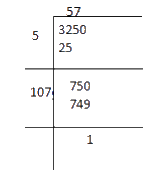The remainder obtained is $$1$$. The square of $$57$$ is less than $$3250$$ by $$1$$. Therefore, the required perfect square  $$= 3250 − 1 = 3249$$

$${\rm{And}}\;\sqrt {3249}= 57$$

(iv)

Square root of $$825$$ can be calculated by the long division method as follows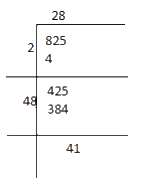The remainder is $$41$$.it shows that the square of $$28$$ is less than $$825$$ by $$41$$.

Therefore, required perfect square $$= 825 − 41 = 784$$

$${\rm{}}\;\sqrt {784}= 28$$

(v)

Square root of $$4000$$ can be calculated by the long division method as follows.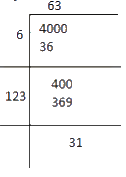The remainder is $$31$$, it represents that the square of $$63$$ is less than $$4000$$ by $$31.$$ Therefore, the square perfect square $$= 4000 − 31 = 3969$$.

$$\sqrt {3969}= 63$$

## Chapter 6 Ex.6.4 Question 5

Find the least number which must be added to each of the following numbers so as to get a perfect square. Also find the square root of the perfect square so obtained.

(i) $$525$$

(ii) $$1750$$

(iii) $$252$$

(iv) $$1825$$

(v) $$6412$$

### Solution

What is known?

Numbers that are not perfect square

What is unknown?

What must be added to the numbers so as to get perfect square

Steps:

(i)

Square root of $$525$$ is calculated by long division method as follows.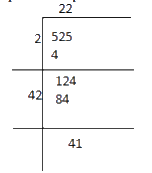It is evident that $${22^2} < 525$$

Next, the perfect square of $$23$$ is

$${23^2} = 529$$

Hence, the number to be added to $$529$$

\begin{align}&= {23^2} - 525\\&= 529 - 525\\&= 4\end{align}

The required perfect square is $$525 + 4 = 529$$

$$\sqrt {529}= 23$$

(ii)

Square root of $$1750$$ can be calculated by the long division method as follows.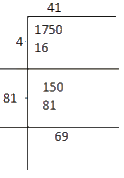The remainder is $$69$$.

This shows that $${41^2} < 1750$$

Next perfect square is $${42^2} = 1764$$

Hence number to be added to $$1750$$

\begin{align}&= {42^2} - 1750\\&= 1764 - 1750\\&= 14\end{align}

The required perfect square is $$1750 + 14 = 1764$$

$$\sqrt {1764}= 42$$

(iii)

Square root of $$252$$ is calculated as follows.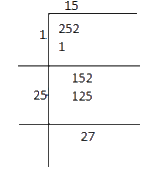The remainder is $$27.$$

This shows that $${15^2} < 252$$

Next perfect square is $${16^2} = 256$$

Hence number to be added to $$252$$

\begin{align}&= {16^2} - 252\\&= 256 - 252\\&= 4\end{align}

The required perfect square is $$252 + 4 = 256$$

$${\rm{}}\;\sqrt {256}= 16$$

(iv)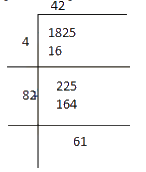Square root of $$1825$$ is calculated as follows.

The remainder is $$61.$$

This shows that $${42^2} < 1825$$

Next perfect square is $${43^2} = 1849$$

Hence number to be added to $$1825$$

\begin{align}&= {43^2} - 1825\\&= 1849 - 1825\\&= 24\end{align}

The required perfect square is $$1825 + 24 = 1849$$

$${\rm{And}}\;\sqrt {1849}= 43$$

(v)

Square root of $$6412$$ is calculated as follows.$${80^2} < 6412$$The remainder is $$12.$$This shows that

Next perfect square is $${81^2} = 6561$$

Hence number to be added to $$6412$$ is

\begin{align}&= {16^2} - 252\\&= 256 - 252\\&= 4\end{align}

The required perfect square is $$6412 + 149 = 6561$$

$${\rm{And}}\;\sqrt {6561}= 81$$

## Chapter 6 Ex.6.4 Question 6

Find the length of the side of a square whose area is $$441\;\rm{m^2}$$ .

### Solution

What is known?

Area of the square

What is unknown?

Length of the side of a square

Reasoning:

Since, area of a square is equals to square of its side.

The length of the side can be calculated by finding the square root of the area.

Steps:

Area of the square = side of a square x side of a square

\begin{align}441\;\rm{m^2} = \text{(side of a square)}^2\end{align}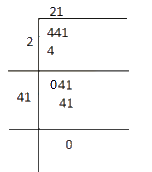$${\text{Side of a square}} = \;\sqrt {441}= 21\;{\rm{m}}$$

## Chapter 6 Ex.6.4 Question 7

In a right triangle $$\rm{}ABC$$, $$\rm{}∠B = 90°$$.

(i) If $$AB = 6 \,\rm{ cm}$$, $$BC = 8\, \rm{cm}$$, find $$AC$$

(ii) If $$AC = 13\,\rm{cm},$$  $$BC = 5 \,\rm{cm}$$, find $$AB$$

### Solution

What is known?

Two sides right triangle

What is unknown?

One side of the given triangle

Reasoning:

In a right-angle triangle if two sides are given then third side can be calculated using the Pythagoras theorem.

Steps:

(i)

$$AB = 6\;\rm{cm}$$ $$BC = 8\;\rm{cm}$$  $$AC=$$ ?

According to Pythagoras theorem

\begin{align}{\rm{A}}{{\rm{C}}^{\rm{2}}}\,{\rm{ }}&= \;{\rm{A}}{{\rm{B}}^{\rm{2}}}\;{\rm{ + }}\;{\rm{B}}{{\rm{C}}^{\rm{2}}}\\{\rm{A}}{{\rm{C}}^{\rm{2}}}\;{\rm{ }}&= \;{{\rm{(6)}}^{\rm{2}}}\;{\rm{ + }}\;{{\rm{(8)}}^{\rm{2}}}\\{\rm{A}}{{\rm{C}}^{\rm{2}}}\;{\rm{ }}&= \;{\rm{100}}\\\;\,{\rm{AC}}\;{\rm{ }}&= \;\sqrt {{\rm{100}}} \\\;\,{\rm{AC}}\;{\rm{ }}&= \;{\rm{10}}\;{\rm{cm}}\end{align}

(ii)

$$AC = 13\;\rm{cm}$$ , $$BC = 5\;\rm{cm}$$ , $$AB$$=?

According to Pythagoras theorem

\begin{align}{\rm{A}}{{\rm{C}}^{\rm{2}}}\;{\rm{ }}&= \;{\rm{A}}{{\rm{B}}^{\rm{2}}}\;{\rm{ + }}\;{\rm{B}}{{\rm{C}}^{\rm{2}}}\\{{\rm{(13)}}^{\rm{2}}}\;{\rm{ }}&= \;{\rm{A}}{{\rm{B}}^{\rm{2}}}\;{\rm{ + }}\;{{\rm{(5)}}^{\rm{2}}}\\{\rm{169}}\;{\rm{ }}&= \;{\rm{A}}{{\rm{B}}^{\rm{2}}}\;{\rm{ + }}\;{\rm{25}}\\ {\rm{A}}{{\rm{B}}^{\rm{2}}}\;{\rm{ }}&= \;{\rm{169}} - {\rm{25}}\;{\rm{ }}=\;{\rm{144}}\\{\rm{AB}}\;{\rm{ }}&= \;\sqrt {{\rm{144}}} \;{\rm{ }}= \;{\rm{12}}\;{\rm{cm}}\end{align}

## Chapter 6 Ex.6.4 Question 8

A gardener has $$1000$$ plants. He wants to plant these in such a way that the number of rows and the number of columns remain same. Find the minimum number of plants he needs more for this.

If number of rows and number of columns are equal then number of plants has to be a perfect square

### Solution

What is known?

Number of plants.

What is unknown?

Minimum number of more plants to make the number of rows and number of columns same.

Reasoning:

If number of rows and number of column are equal then number of palant has to be a prefect square.

Steps:

Number of plants $$= 1000$$

The square root of $$1000$$ can be calculated by long division method.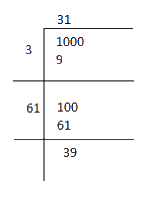The remainder is $$39.$$

It shows that $${31^2} < 1000$$

The square of $$32$$ is $$1024$$

Hence number to be added to $$1000$$ to make it perfect square

.\begin{align} &= {32^2} - 1000\\ &= 1024 - 1000\\ &= 24 \end{align}

Thus, the required number of plants $$=24$$

## Chapter 6 Ex.6.4 Question 9

There are $$500$$ children in a school. For a P.T. drill they have to stand in such a manner that the number of rows is equal to number of columns. How many children would be left out in this arrangement?

Here, we have to find the number which should be subtracted from total number of children to make it a perfect square.

### Solution

What is known?

Number of children in a school.

What is unknown?

Number of children would be left out in PT drill.

Reasoning:

Here, we have to find the number which should be subtracted from total number of children to make it a prefect square.

Steps:

Number of children in a school $$= 500$$ The square root of $$500$$ can be calculated using long division method.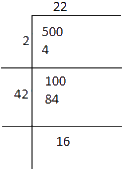The remainder is $$16$$. It shows that $${(22)^2}$$ is less than $$500$$.

Therefore, perfect square can be obtained by subtracting $$16$$ from the given number.

Therefore, required perfect square is

\begin{align}&= 500 - 16\\&= 484\end{align}

$${\rm{And}}\;\sqrt {484}= 22$$

Number of children left out in PT drill arrangement $$= 16$$

Related Sections
Related Sections
Instant doubt clearing with Cuemath Advanced Math Program